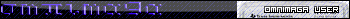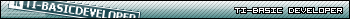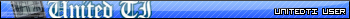###Author Topic: Physics Lessons  (Read 43836 times)

0 Members and 1 Guest are viewing this topic.

####meishe91

• Super Ninja
• LV11 Super Veteran (Next: 3000)
••• Posts: 2946
• Rating: +115/-11##### Re: Physics Lessons
« Reply #15 on: March 28, 2010, 05:20:46 am »
Hey Builderboy, how is the next section of this looking? Just curious because I can't wait to learn moreSpoiler For Spoiler:For the 51st time, that is not my card! (Magic Joke)

#### SirCmpwn

• Guest##### Re: Physics Lessons
« Reply #16 on: March 28, 2010, 12:11:28 pm »
Builderboy, amazing tutorial.
I would like to suggest however that this be discussed more in the frame of inertia, because applying inertia takes care of this and allows for other movements beyond gravity to be more accurately portrayed.

####DJ Omnimaga##### Re: Physics Lessons
« Reply #17 on: March 28, 2010, 12:40:03 pm »
maybe also explain to non visual people special vocabulary used in the tutorial for physics. I notice that when I read a tutorial, if I have to go on Wikipedia/Google every sentence, it gets annoying and I eventually lose interest because I can't stay into the tutorial.

####ztrumpet##### Re: Physics Lessons
« Reply #18 on: April 02, 2010, 01:11:37 pm »
*Bump*

Builderboy, when's the next section of this coming?
I know I, for one, am really looking forward to it!If I'm wrong, please correct me!
Unfinished Projects:
 Elmgon 14% Basic Movement Demo Homescreen Game Pack 80% Basic Latest Release Cube Droid Saves the Galaxy 65% Axe Demo Detonate 70% Axe
Completed Projects:
Exodus | Midnight |Drifter | Axe Snake | Jump! | Factory Theta | Spider | Plot Drop | Papi Jump | Numb3rs | Nibbler | Boost | Duel Tile Map Editor | Homescreen Map Editor | Key Group Check | Oasis

####Raylin

• Godslayer
• LV10 31337 u53r (Next: 2000)
••• Posts: 1392
• Rating: +83/-25
• I am a certifiable squirrel ninja.##### Re: Physics Lessons
« Reply #19 on: April 02, 2010, 01:30:03 pm »
^++
Agreed and seconded.

Sarah: TI-83 Plus Silver Edition [OS 1.19]
Cassie: TI-86 [OS 1.XX]
Elizabeth: TI-81 [OS 1.XX]
Jehuty: TI-83 Plus Silver Edition [OS 1.19]
Tesla: CASIO Prizm####Builderboy##### Re: Physics Lessons
« Reply #20 on: April 02, 2010, 06:20:31 pm »
Its coming soon-ish.  School has suddenly gone into overdrive with teachers starting to prepare for AP testing, so its been pretty hectic X.X Collision is being worked on tho, as well as an update to gravity####DJ Omnimaga##### Re: Physics Lessons
« Reply #21 on: April 02, 2010, 07:35:00 pm »
I hope they won't overload you even more with projects, it would suck if you had to stop working on this. Good luck on everything!

####meishe91

• Super Ninja
• LV11 Super Veteran (Next: 3000)
••• Posts: 2946
• Rating: +115/-11##### Re: Physics Lessons
« Reply #22 on: April 02, 2010, 08:47:30 pm »
Ya, I know what you mean about the AP test prep time. Glad to hear its coming though.
Spoiler For Spoiler:For the 51st time, that is not my card! (Magic Joke)

####Builderboy##### Re: Physics Lessons
« Reply #23 on: April 07, 2010, 01:23:39 am »
OK here is a quick (kinda) lesson on trig and the wonders of Triangles!  Unplanned, so its coming before collisions************
Trigonometry    NOTE: put your calc in degrees mode!
************

Trig is one of those things that can be very usefull in games, but is actualy fairly
difficult to figure out without some good instruction.  That is where this tutorial
is going to try to help.

trigonometry is the mathematics of trianlges.  Take this triangle for instance

Code: [Select]
       |\      | \  a   |  \ C    (hypotenuse)(leg) |   \      |    \      +-----         b (leg)
It is a right triangle because the angle between side A and side B is 90 degrees.  This is
the only type of trignale we will discuss in this tutorial, and usualy it is the only one you
will need.

In addition to having 3 sides, there are also 3 angles. AB, AC, and BC.  You can also call them
by the sides that are *opposite* to that angle, so angle AB would be angle C.  We already
established that angle AB is 90 degrees.  This triangle is a RIGHT triangle, because it has one
right (90 degree) angle, and Right Triangles are your friendFor starters, here is the pythagorean theorem:

A^2 + B^2 = C^2

Hmmm interesting, for all Right Trignales, the sum of each leg squared, equals the hypotenuse squared.
(remember, the legs are the two sides that make the right angle)  What this means for us is that
for any right triangle, if we are given 2 sides, we can find the other with this equation.  This
is a way to calculate the distance to a point, as you can use the 2 legs as the X and Y distances
to a point.

Code: [Select]
               B              /|     / |    The distance from A to B is the hypotenuse of a right triangle, with X and Y    /  |    as the legs.   /   | Y  /    |    Distance^2 = X^2 + Y^2     Take the square root, and we have: /     | A------+    Distance = SQRT(X^2 + Y^2)             X
Now, we have worked with the sides, what about the angles?  The angles between two sides is the ammount
of rotation between them:

Code: [Select]
  |  |          \                         /  |           \                       /  |            \                     /   | 90 degrees  \   135 degrees     /  45 degrees   |              \                 /  +---------      --------        ---------
Somtimes we want to rotate a sprite or an object around a point.  Like this:

Code: [Select]
ZStandardZIntegerAxesOffClrDrawFor(F,0,360,5Pt-On(29cos(F),29sin(FEnd
What??? How did i just do that? Well the answer lies in trig, and in right triangles.  You may not think
that triangles have much to do with circles, but in fact, they have everything to do with eachother.
A circle contains all the points a certain radius from a center point.  So given a radius, there are
different X,Y points that lie onto a circle.  Does this look farmiliar?  Look again at the distance formula:

Distance = SQRT(X^2 + Y^2)

If we say that Distance is the radius, this distance equation becomes the equation of a circle!  :O
It gives us an euqation, where if we have a radius (distance) and an X or Y value, we can find the
other coordinate.

Try solving the distance equation for Y

Distance = SQRT(X^2 + Y^2)
Distance^2 = X^2 + Y^2
Distance^2 - X^2 = Y^2
SQRT(Distance^2 - X^2) = Y

Y = SQRT(Distance^2 - X^2)

Try putting this into Y1 in your calculator, set Distance to 5 lets say, and graph it!  You get a circle!
(or half of one anyway... stupid functions...)

Wow, thats really cool, we can get a circle without doing any trig at all!

Now comes the final challenge, using the above equation, we can find all of the points on a circle, given
the radius, but what if we want a singe point?  We already have the radius, but we do not have any other

variables
to determine which point to use.  We need another, and that is the angle.  A circle goes accross 360 degrees
or angle, starting at the rightmost point at 0 degrees and rotating accross the circle counterclockwise until
it gets to 360 degrees, where it started.

Now it is time to introduce Sin, Cos, and Tan.  These functions pertain to triangles and their angles, and are
all very usefull.  For right triangles, you only take the sin, cos, or tan of angles that are NOT the Right

Angle
So for this triable they would be AC and BC

Code: [Select]
       |\ Sin of an angle equals the opposite leg over the hypotenuse      | \ Sin(BC) = A/C Sin(AC) = B/C  a   |  \ C    (hypotenuse) Cos of an angle equals the adjecent leg over the hypotenuse(leg) |   \ Cos(BC) = B/C Sin(AC) = A/C      |    \ Tan of an angle equals the opposite over the adjecent      +----- Tan(BC) = A/B Tan(AC) = B/A         b (leg)
These trigonometric equations give us some interesting power.  If we know Hypotenuse C and Angle BC,
we can find leg A.

Sin(BC) = A/C
Sin(BC)*C = A
A = Sin(BC)*C

And we can also find leg B as well

Cos(BC) = B/C
Cos(BC)*C = B
B = Cos(BC)*C

Do you know what this meants?  Given a distance, and an angle, we can find the coordinates of that point!

Code: [Select]
               B              /|     / |      /  |       /   | Y  /    |   /     | A------+                X
Given the angle at A, and the distance AB, we can calculate the distances X and Y, which are the coordinates of
Point B.

X = R*Cos(A)
Y = R*Sin(B)

its that simple!  And for all angles, point B will be a distance of R form the center, which is the
*exact* definition of a circle!  Given an angle and a radius, using these two equations we can find a
specific point on a circle.

This program shows how the triangles fit into the circle as it travels around the circle:

Code: [Select]
ZStandardZIntegerAxesOffClrDrawDegree0->FCircle(0,0,30While 1Line(0,0,A,B,0 //hypotenuseLine(0,0,A,0,0 //right legLine(A,0,A,B,0  //left leg29Cos(F)->A //find the coordinates of the next point on the circle29Sin(F)->BLine(0,0,A,BLine(0,0,A,0Line(A,0,A,BF+5->FEnd
« Last Edit: November 18, 2012, 04:14:48 am by Builderboy »

####DJ Omnimaga##### Re: Physics Lessons
« Reply #24 on: April 07, 2010, 02:56:08 am »
nice tutorial, I didn't read it all, but I am getting some of it atm. Thanks for posting this. I haven't done trig in 8 years now and we only did it for a week at mostat school But I ddint take the hardest math classes, so...

####meishe91

• Super Ninja
• LV11 Super Veteran (Next: 3000)
••• Posts: 2946
• Rating: +115/-11##### Re: Physics Lessons
« Reply #25 on: April 07, 2010, 03:26:21 am »
Very nice. I'll probably read it again tomorrow since it's so late right now but it's good.
I would like to point out to everybody though that your calculator must be set in Degrees mode, not Radians, to follow this. You can follow it in Radians if you know the proper conversions but that's just more work than you need to doSpoiler For Spoiler:For the 51st time, that is not my card! (Magic Joke)

#### SirCmpwn

• Guest##### Re: Physics Lessons
« Reply #26 on: April 07, 2010, 10:17:37 am »

####DJ Omnimaga##### Re: Physics Lessons
« Reply #27 on: April 07, 2010, 10:50:03 am »
Nice. It appears to erase parts of the circle, though, so you might want to store it in a pic and recall it every frame. Also, instead of Circle(X,Y,radius do Circle(X,Y,radius,{i where i is the imaginary i, not the lowercase ALPHA i. This will be slightly less accurate, but be 3 times faster

#### SirCmpwn

• Guest##### Re: Physics Lessons
« Reply #28 on: April 07, 2010, 11:28:28 am »
^Explain.  What is the whole {i thing?  And also, for the purposes of a tech demo, I think the parts of the circle can probably stay erased.

####DJ Omnimaga##### Re: Physics Lessons
« Reply #29 on: April 07, 2010, 11:50:25 am »
i

EDIT: oops accidentally hit a button or somethingThere we go http://tibasicdev.wikidot.com/circle

It's basically fast circles. Before this was discovered in 2006 people relied on ASM libs to display those.
« Last Edit: April 07, 2010, 11:51:46 am by DJ Omnimaga »